# Models available in the COVOID package

Brief descriptions of the types of models provided by the COVOID package.

Oisin Fitzgerald https://cbdrh.med.unsw.edu.au/people/mr-oisin-fitzgerald (Centre for Big Data Research in Health, UNSW Medicine)https://cbdrh.med.unsw.edu.au , Mark Hanly https://cbdrh.med.unsw.edu.au/people/mr-mark-hanly (Centre for Big Data Research in Health, UNSW Medicine)https://cbdrh.med.unsw.edu.au , Tim Churches https://timchurches.github.io/blog/ (South Western Sydney Clinical School, UNSW Medicine)https://swscs.med.unsw.edu.au
2020-05-30

## Overview

Each model is associated with an initial state, parameter and simulation function. If you are using a model with the code * we pass the parameters for the ODE system to the `*_param` function, the initial compartment states to `*_state0` and finally run the system using `simulate_*`. Models named `*_c` are age-structured, and require a contact matrix and age distribution. These are also the most mature models, all models come with a lifecycle badge making clear the potential for future changes.

## Homogeneous mixing

These models assume the contact rates between individuals in the population can be adequately described by a single value, rather than varying by age or setting.

### SIR model

The key functions for this model are `sir_state0` `sir_param` and `simulate_sir`.

Figure 1: SIR model forward dynamics

Figure 2: SIR model infection dynamics

``````
# A minimal example
param <- sir_param(R0 = 2.5,gamma = 0.1)
state0 <- sir_state0(S = 100,I = 1,R = 0)
res <- simulate_sir(t = 100,state_t0 = state0,param = param)
plot(res,y=c("S","I","R"),main="SIR model")``````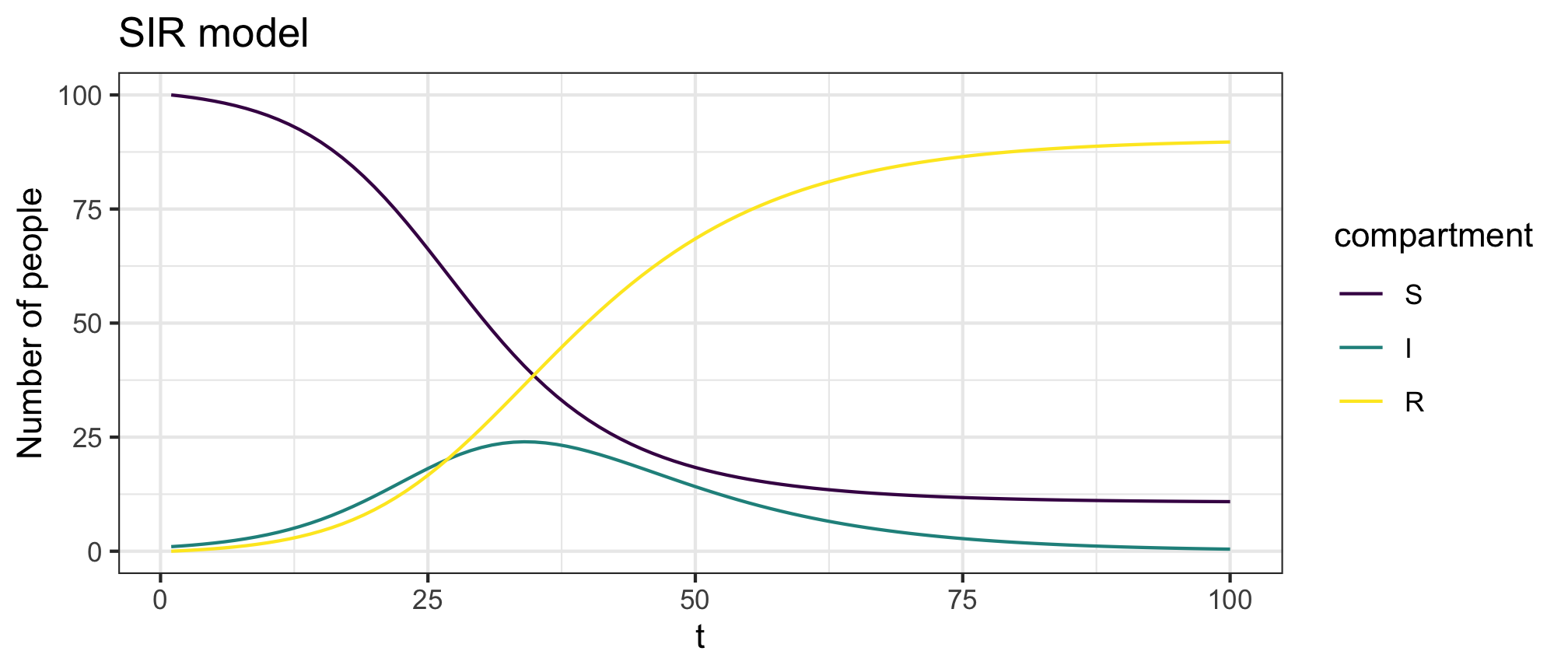### SEIR model

The key functions for this model are `seir_state0` `seir_param` and `simulate_seir`.

Figure 3: SEIR model forward dynamics

Figure 4: SEIR model infection dynamics

``````
# A minimal example
param <- seir_param(R0 = 2.5,gamma = 0.1,sigma=0.1)
state0 <- seir_state0(S = 100,E = 1, I = 0,R = 0)
res <- simulate_seir(t = 200,state_t0 = state0,param = param)
plot(res,y=c("S","E","I","R"),main="SEIR model")``````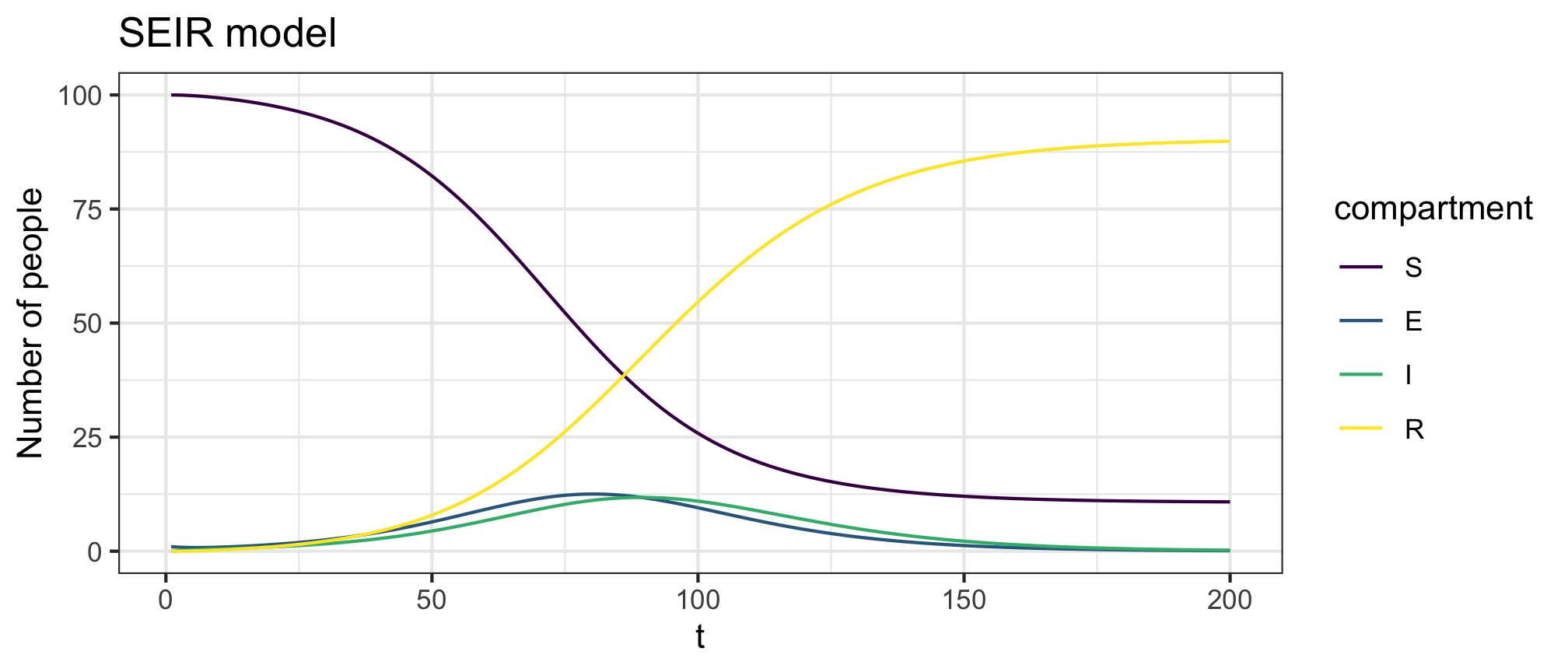### Expanded SEIR model I

This model is recommended for modelling COVID-19, it is based on 1 and 2.

The key functions for this model are `seir1_state0` `seir1_param` and `simulate_seir1`.

Figure 5: Expanded SEIR model I forward dynamics

Figure 6: Expanded SEIR model I infection dynamics

``````
# A minimal example
state0 <- seir1_state0(S = 1e5, E1 = 90, E2 = 40)
param <- seir1_param(R0=2.5,sigma1=0.2,sigma2=0.2,gamma1=0.2,gamma2=0.2,gamma3=0.2,
Qeff=0.5,Heff=0.9,rho=0.1,alpha=0.1,eta=0.02)
res <- simulate_seir1(t = 250,state_t0 = state0,param = param)
plot(res,y=c("S","E","I","Recov","Fatal"),main="Expanded SEIR model I")``````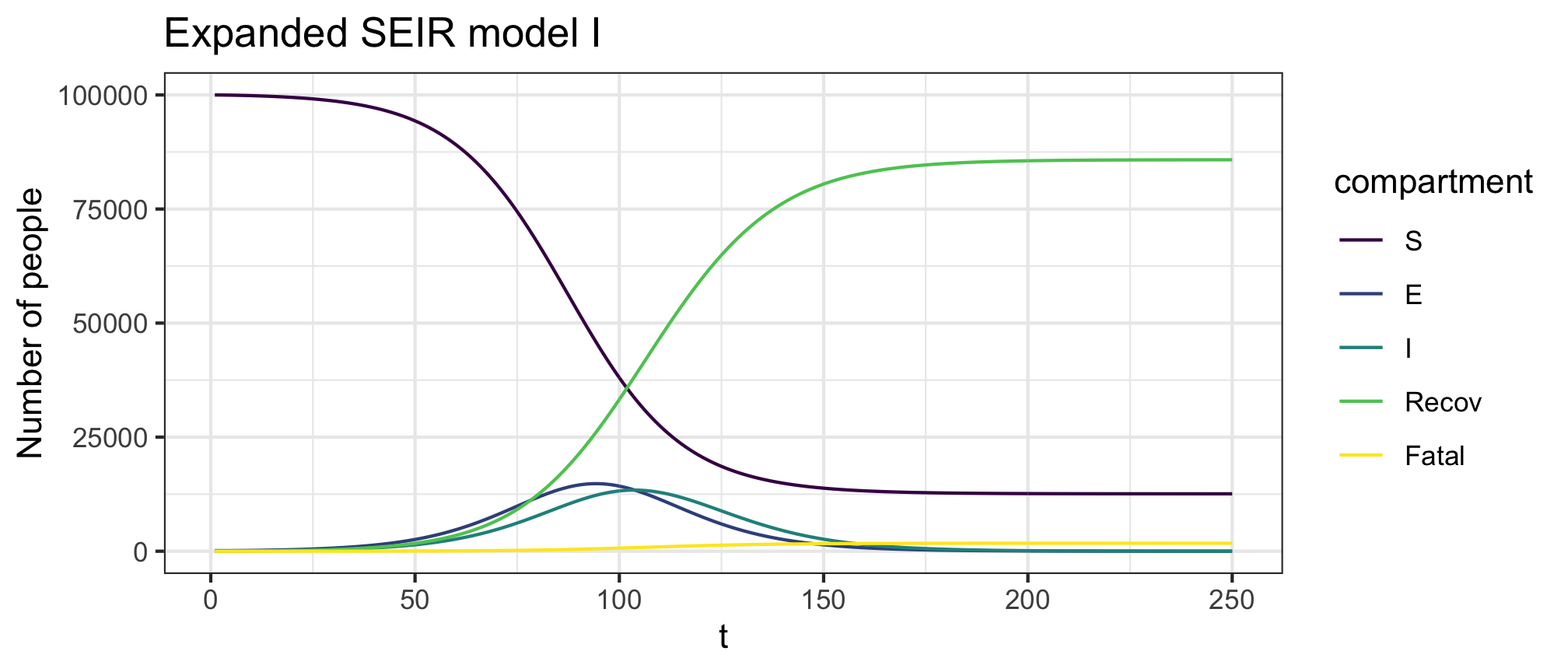### Expanded SEIR model II

This model is recommended for modelling COVID-19, it is a (partial) reproduction of 2.

The key functions for this model are `seir2_state0` `seir2_param` and `simulate_seir2`.

Figure 7: Expanded SEIR model II (Doherty model) forward dynamics

Figure 8: Expanded SEIR model II (Doherty model) infection dynamics

``````
# A minimal example
state0 <- seir2_state0(S = 1e5, E1 = 90, E2 = 40)
param <- seir2_param(R0=2.5,lambdaimp=0,sigma1=0.2,sigma2=0.2,gamma1=0.2,gamma2=0.2,
gammaq1=0.1,gammaq2=0.1,Qeff=0.5,Meff=0.99,rho=0.0,eta=1/sqrt(2),
alphamBeta=0.5,probHospGivenInf=0.09895,delta=1/14,kappa=20,pm=1)
res <- simulate_seir2(t = 250,state_t0 = state0,param = param)
plot(res,y=c("S","E","I","Recov","Fatal"),main="Moss et al (2020) model")``````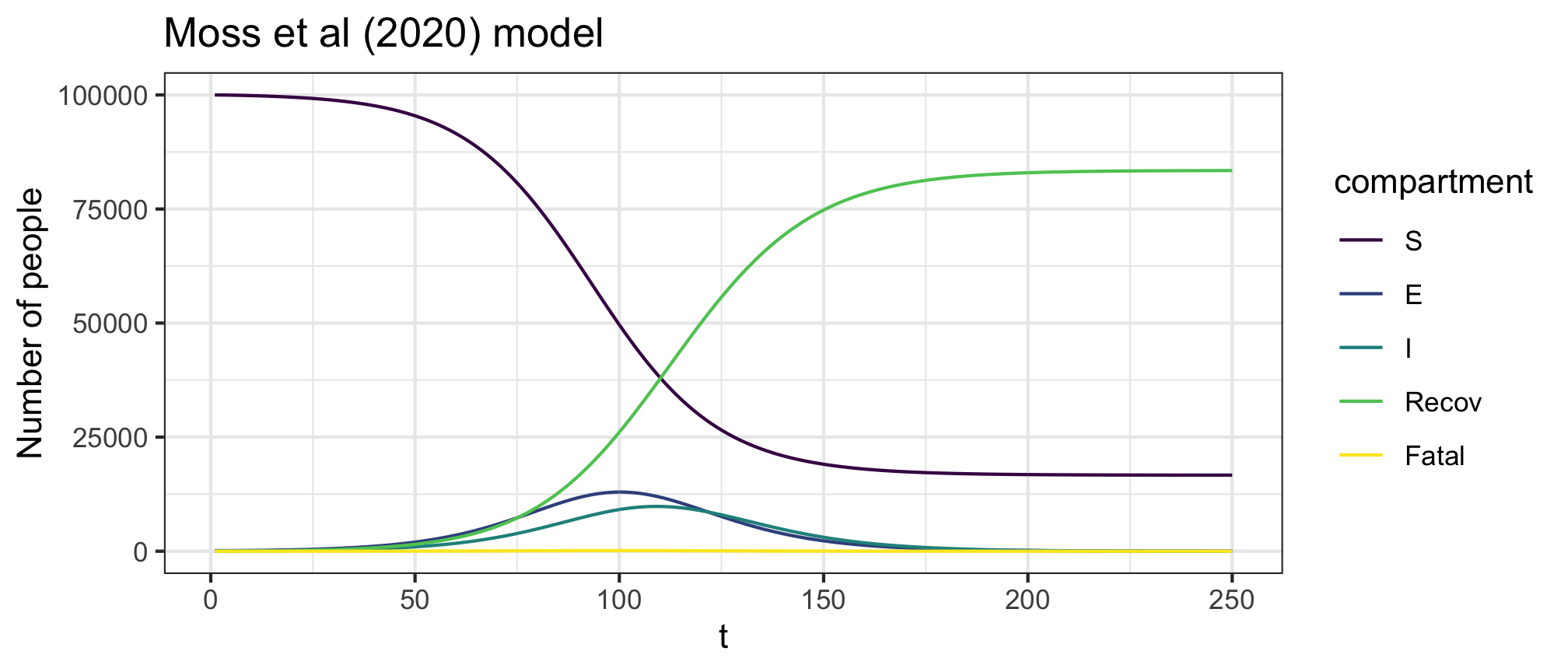## Age structured mixing

These models structure the population by discrete age partitions, with the contact rate assumed to be age and setting dependent (see figure).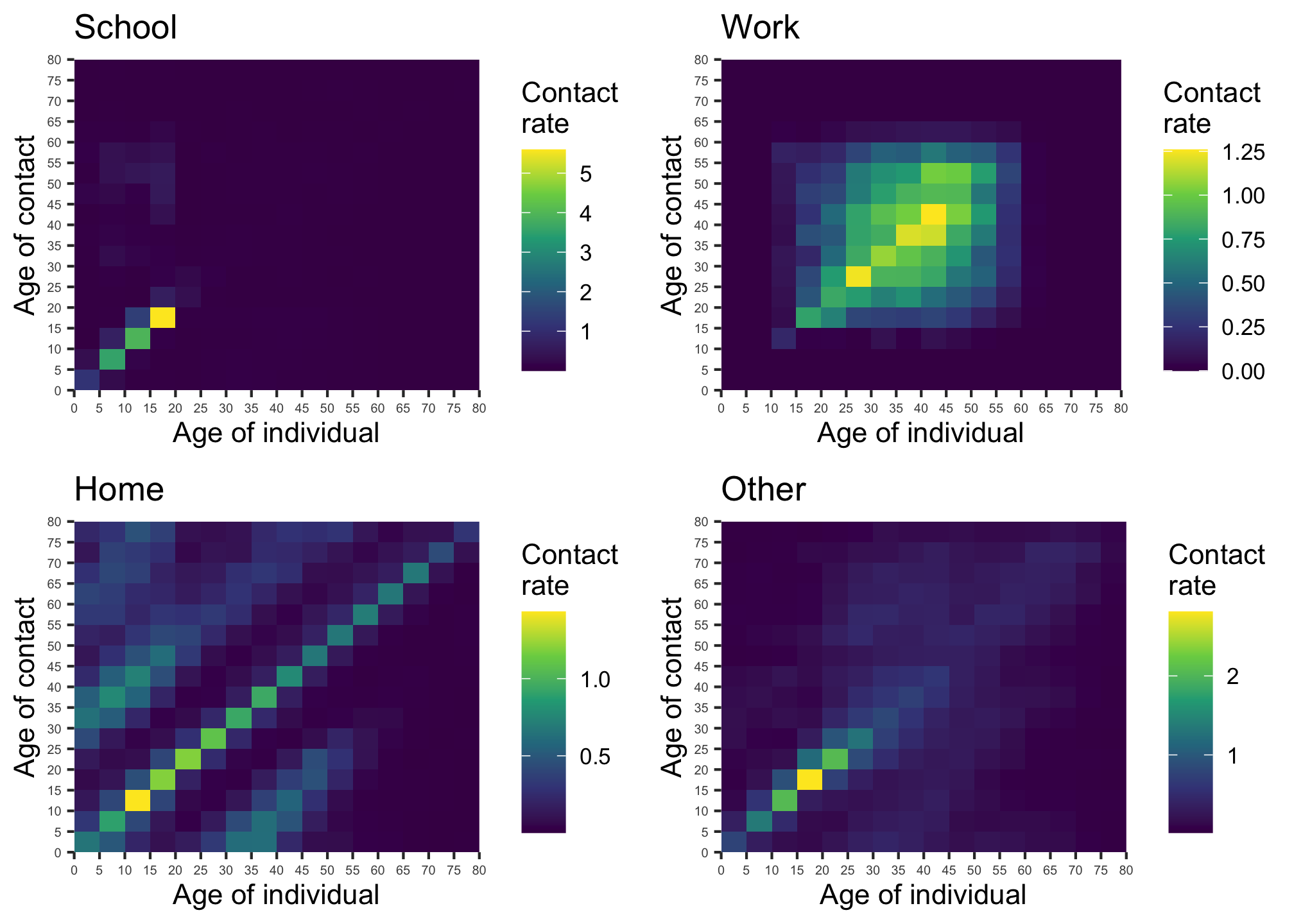Figure 9: Contact matrices for school, work, home and other locations (source: Prem, Cook and Jit (2017)). Note the differing scales.

### SIR model

The key functions for this model are `sir_c_state0` `sir_c_param` and `simulate_sir_c`.

Figure 10: Age structured SIR model forward dynamics. There are j=1,…,J age groups.

Figure 11: Age structured SIR model infection dynamics. There are j=1,…,J age groups.

``````
# A minimal example
cm_oz <- import_contact_matrix("Australia","general")
dist_oz <- import_age_distribution("Australia")
param <- sir_c_param(R0 = 2.5,gamma = 0.1,cm=cm_oz,dist=dist_oz)
nJ <- ncol(cm_oz)
S <- rep(100,nJ)
I <- rep(1,nJ)
R <- rep(0,nJ)
state0 <- sir_c_state0(S = S,I = I,R = R)
res <- simulate_sir_c(t = 150,state_t0 = state0,param = param)
plot(res,y=c("S","I","R"),main="Age structured SIR model")``````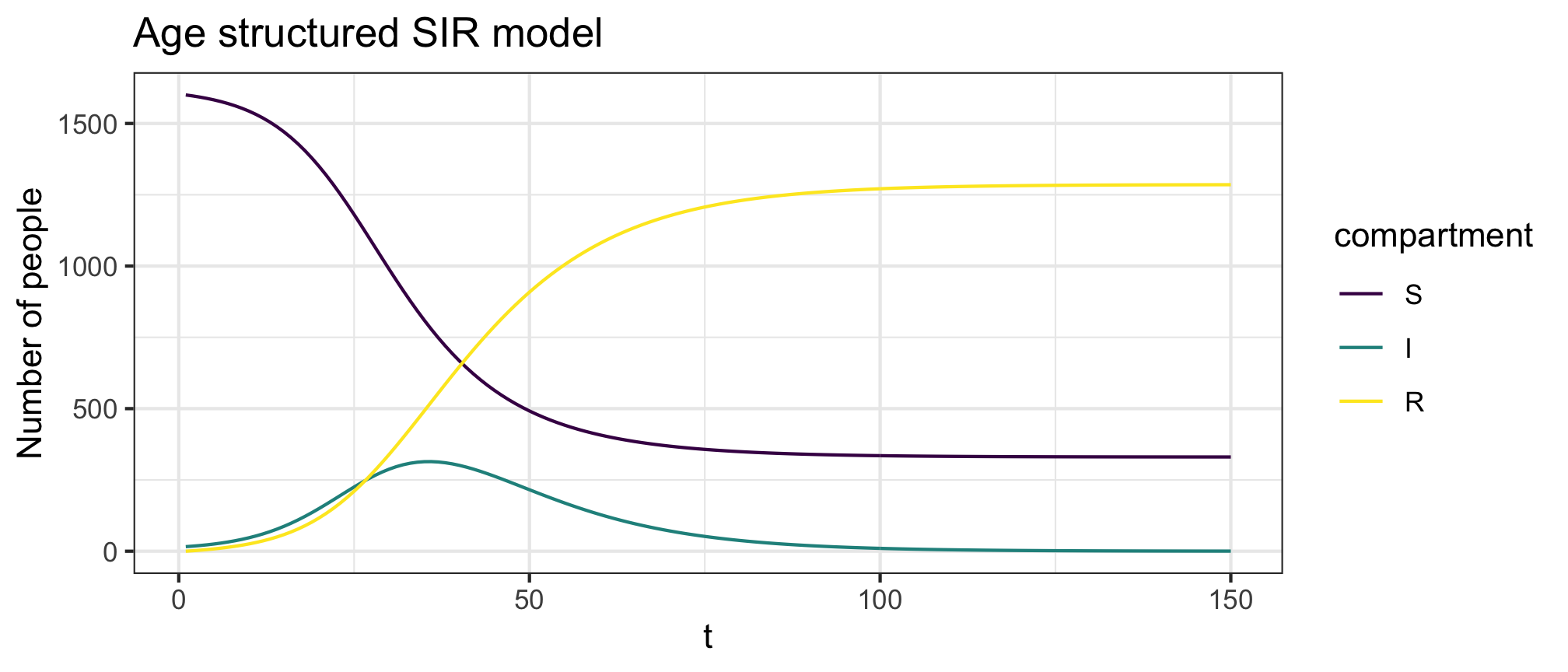### SEIR model

The key functions for this model are `seir_c_state0` `seir_c_param` and `simulate_seir_c`.

Figure 12: Age structured SEIR model forward dynamics. There are j=1,…,J age groups.

Figure 13: Age structured SEIR model infection dynamics. There are j=1,…,J age groups.

``````
# A minimal example
cm_oz <- import_contact_matrix("Australia","general")
nJ <- ncol(cm_oz)
dist_oz <- import_age_distribution("Australia")
S <- rep(1000,nJ)
E <- rep(1,nJ)
I <- rep(0,nJ)
R <- rep(0,nJ)
state0 <- seir_c_state0(S = S,E = E,I = I,R = R)
param1 <- seir_c_param(R0 = 2.5,sigma=0.1,gamma = 0.1,cm=cm_oz,dist=dist_oz)
res1 <- simulate_seir_c(t = 200,state_t0 = state0,param = param1)
plot(res1,y=c("S","E","I","R"),main="Age structured SEIR model")``````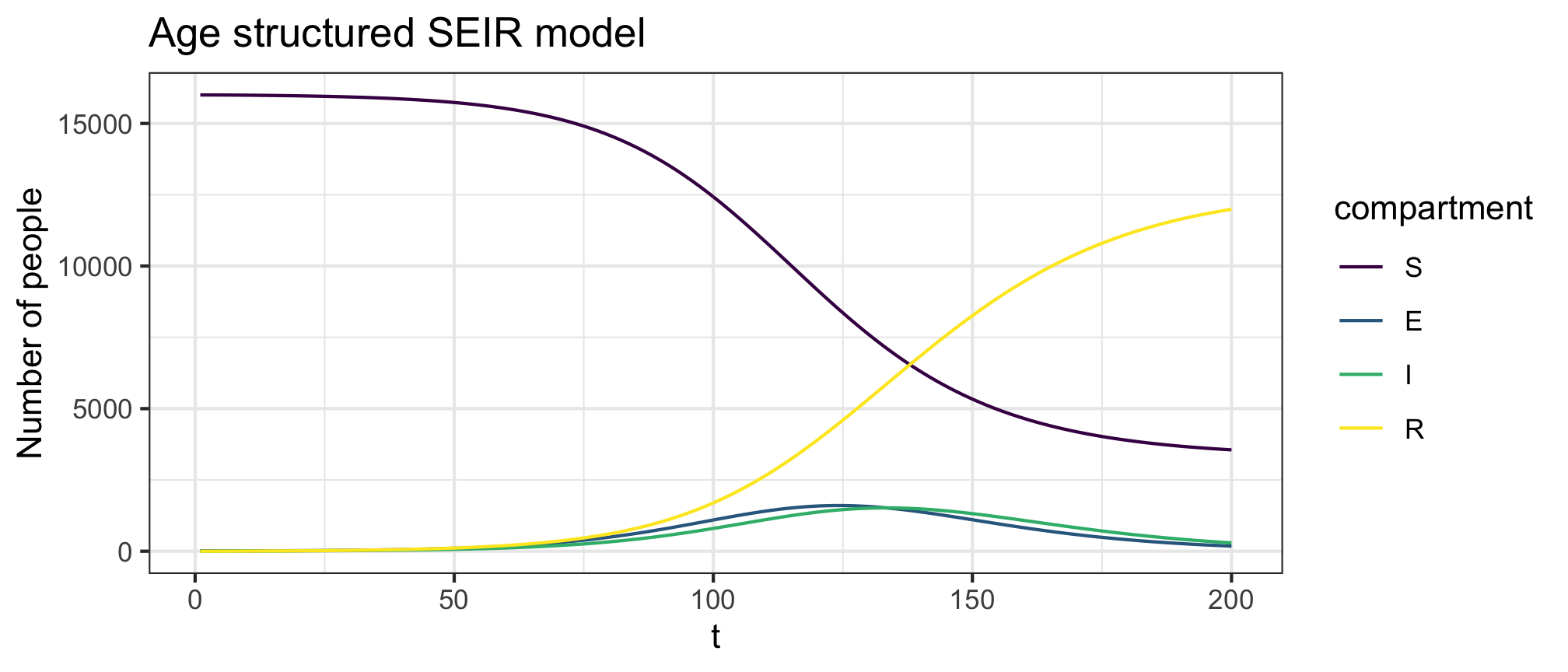## Acknowledgments

All graphs created using DiagrammR

1. Churches T, Jorm L. COVOID: A flexible, freely available stochastic individual contact model for exploring covid-19 intervention and control strategies (preprint). JMIR Preprints. Published online 2020.

2. Moss R, Wood J, Brown D, et al. Modelling the impact of covid-19 in australia to inform transmission reducing measures and health system preparedness. medRxiv. Published online 2020.

### Corrections

If you see mistakes or want to suggest changes, please create an issue on the source repository.

### Reuse

Text and figures are licensed under Creative Commons Attribution CC BY 4.0. Source code is available at https://github.com/CBDRH/covoid, unless otherwise noted. The figures that have been reused from other sources don't fall under this license and can be recognized by a note in their caption: "Figure from ...".

### Citation

`Fitzgerald, et al. (2020, May 30). COVOID: Models available in the COVOID package. Retrieved from https://cbdrh.github.io/covoidance/COVOID-available-models.html`
```@misc{fitzgerald2020d,